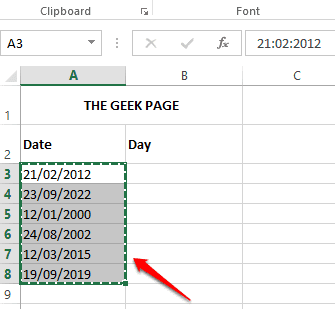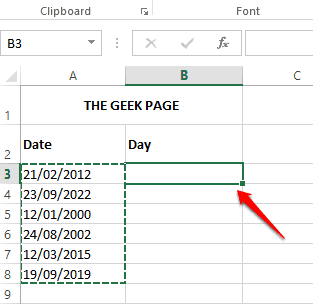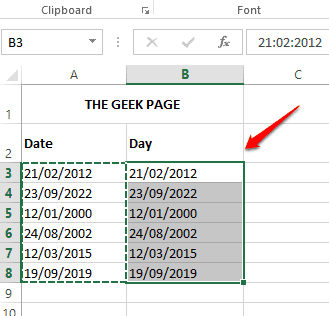# How to Extract Day Values from Date Values in Microsoft Excel

In some scenarios, you might want to extract the day from a date. Let’s say you have the date 27/05/2022 and there should be a way your Excel sheet should be able to tell you that it’s a Friday. If this can be made practical, it could find applications in many cases such as finding the workdays of a project, on school assignments, etc.

Here in this article, we have come up with 3 different solutions using which you can easily extract the day value from a date value and apply the same for an entire column automatically. Hope you enjoy the article.

## Example Scenario

We have one column named Date which has date values. Another column Day is empty and its values are to be populated by figuring out the day values from the corresponding date values from the Date column.

## Solution 1: By Using Format Cells Options

This is the simplest of all the methods as it doesn’t involve any formulae.

Step 1: Copy the entire date values from column 1, the Date column.

You can either select the whole data, right click, and then choose the Copy option or you can simply press the keys CTRL + C after selecting the data.Step 2: As next, click once on the first cell of the column where you want the Day values to be extracted to.Step 3: Now press the keys CTRL + V to paste the data.Step 4: Right click anywhere on the selected range of cells and click on the option named Format Cells.Step 5: In the Format Cells window, click on the Number tab first. As next, under Category options, click on the Custom option.

Now, under the Type field, type in ddd. Right above the Type field, under the Sample section, you will be able to view the sample data. Because we have given ddd, the sample data displays weekday in 3 letters format, which is Tue in the following example.

Hit the OK button once you are done.If you want the full weekday to be displayed, then instead of ddd, you need to give dddd under the Type field. So that the sample data shows Tuesday.Step 6: That’s it. You have successfully extracted day values from date values just as simple as that.## Solution 2: By Using TEXT Formula Function

This is another simple method using which you can quickly extract the day value from the date value. This method makes use of a formula function named Text().

Step 1: Double click on the first cell of the column where you want the day values to be extracted. Now, copy and paste the following formula onto it.

`=TEXT(A3,"ddd")`

You have to replace A3 with your required cell id that has the date value. Also, ddd would give the day in 3 letter format. For eg., Tue.If you want the day value in full format, for example, Tuesday, then your formula should have dddd as its second argument instead of ddd.Step 2: If you press elsewhere or hit the Enter key, you can see that the formula is successfully applied and that the day value is now successfully extracted.

If you would like to apply the formula across the column, simply click and drag the small square shape present at the bottom right corner of the cell.Step 3: You now have the day values for all the date values present in your Excel sheet.## Solution 3: By Combining CHOOSE and WEEKDAY Formula Functions

This method is relatively complex compared to the first 2 methods listed in this article. This method extracts day values from date values by combining 2 formula functions namely Choose() and Weekday(). However, this method is a little geeky but we love geeky things. Hope you do too.

Step 1: Double click on the first cell of the Day column to edit its contents. Copy and paste the following formula onto it.

`=CHOOSE(WEEKDAY(A3),"SUNDAY","MONDAY","TUESDAY","WEDNESDAY","THURSDAY","FRIDAY","SATURDAY")`

A3 in the formula has to be replaced with the cell id of the cell that has the date value from which you want to extract the day value.## Formula Explanation

The part that gets resolved first in the above formula is WEEKDAY(A3). The Weekday function takes in a date as an argument and returns the weekday in the form of an integer. By default, the function assumes that the week goes from Sunday to Saturday. So, if it’s a normal scenario, you can give just one argument for this function. In this formula, I have given only one argument, A3, which is the cell id of the cell that contains the date from which I need to find the day. But if your week starts from Monday, then you need to give one more argument to your function making it WEEKDAY(A3,2). So your final formula, in that case, would look as shown below.

`=CHOOSE(WEEKDAY(A3,2),"SUNDAY","MONDAY","TUESDAY","WEDNESDAY","THURSDAY","FRIDAY","SATURDAY")`

So WEEKDAY() function returns the weekday of the week in number format. That is, if the day is Tuesday, then WEEKDAY() would return 3.

The CHOOSE() function returns a string from its list of arguments based on its first argument.

CHOOSE() function can have many arguments. The first argument is the index number. The arguments after the index number are the strings that need to be picked based on the index number. For example, if the first argument of CHOOSE() function is 1, then the first string in the list would be returned by the CHOOSE() function.

So in this particular scenario, WEEKDAY() function returns 3 as its return value. Now CHOOSE() function takes this 3 as its first argument. And returns the 3rd string in the list as per this index value. So CHOOSE() function would finally return TUESDAY.

If you would like to return the short format of days, you can make the adjustments in the main formula as shown below.

`=CHOOSE(WEEKDAY(A3,2),"Sun","Mon","Tue","Wed","Thu","Fri","Sat")`

Step 2: If you hit the Enter key or click anywhere else, you can see that the day value is successfully populated in the cell you selected.

To apply the formula across all the cells in the column, click on the small square icon at the corner of the cell and drag it down.Step 3: The formula is now successfully applied to all the cells and the day values are now populated in the entire column.Drop us a comment saying which method is your pick! Please do come back for more amazing Excel articles and for a lot more!